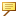Piping > Piping > Setting Up Piping Library > Bend Tables > Bend Table Data in Spec-Driven Piping Mode
Bend Table Data in Spec-Driven Piping Mode
The table data (A) represents the developed bend lengths or allowances (with the presence of the CONVERSION formula) for specific bend radii and pipe outer diameters (T). The table data and the conversion equation are used together, so make sure that the two together give you the correct results.
Observe the following points when using bend table data:
Create your bend tables for 90–degree bends. The values other than 90 degrees are multiplied by A/90, where A represents the specific bend angle in degrees.
You do not have to insert a bend allowance value (A) in every cell in a bend allowance table.
If you leave a particular cell blank, the system uses interpolation to calculate the length value.
For bend radii and outside diameters (OD) between the values in adjacent cells, a linear interpolation is used.The values in the bend tables are independent of the model units. They do not change if you change the model units.
For example, you originally create a pipe assembly with the model units set to inches. The outside diameter is 0.25 and the bend radius is 0.5. The system looks up the bend table to find the developed length at the ordinates (0.25, 0.5). If you change the model units to centimeters and keep the model the same size, the pipe OD becomes 0.625 and the bend radius 1.270. The system looks up the table to find the developed length at the ordinates (0.625, 1.270).